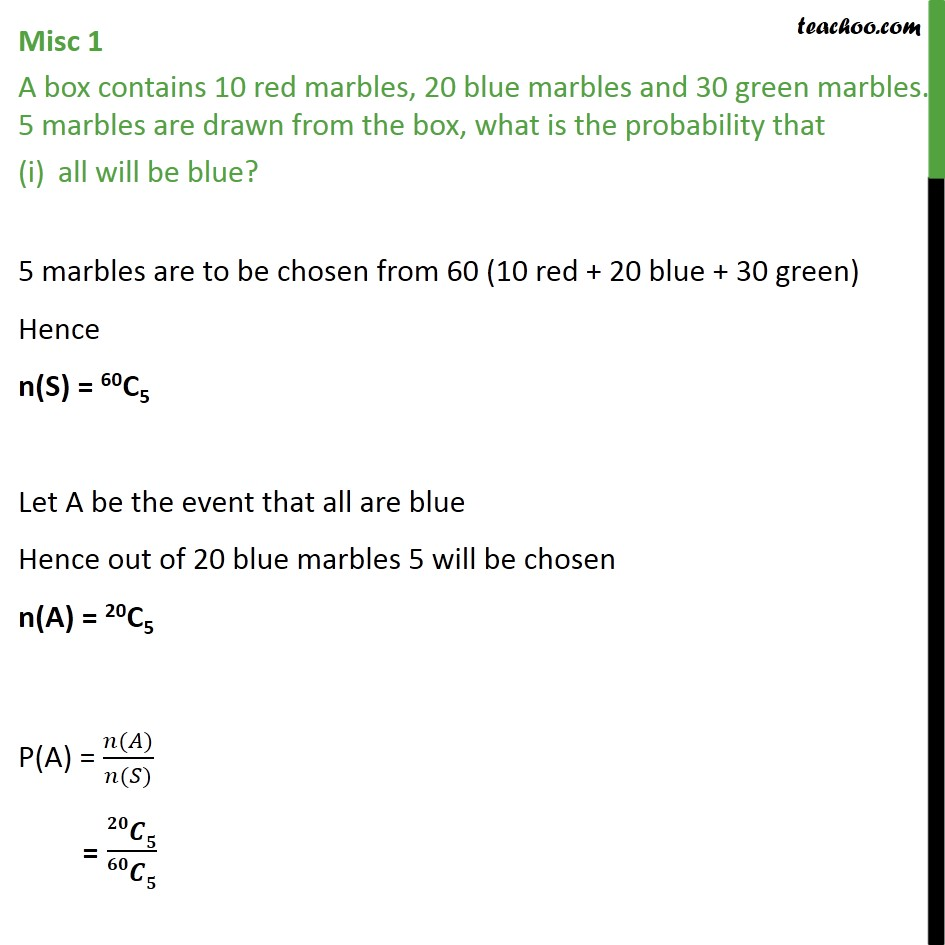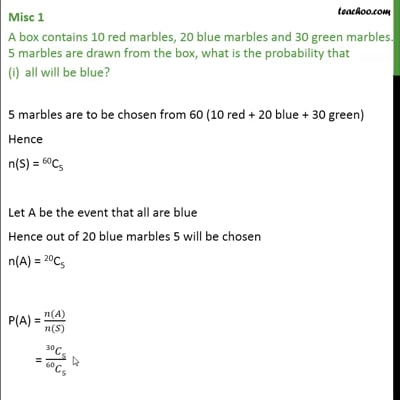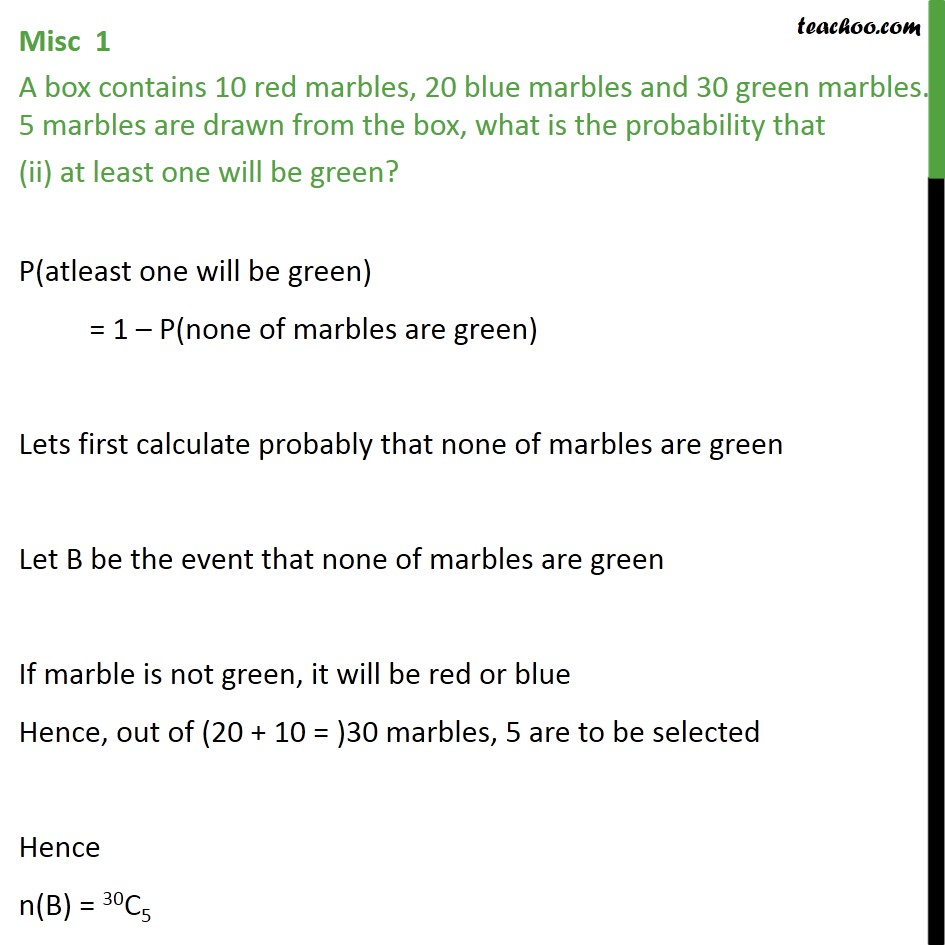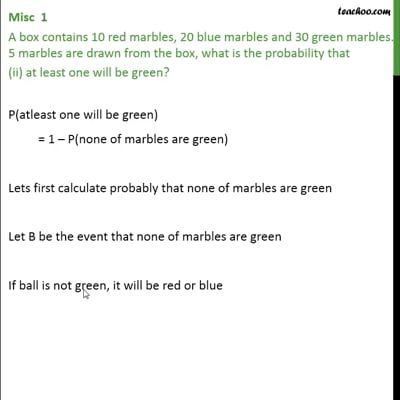Miscellaneous

Chapter 16 Class 11 Probability (Term 2)
Serial order wiseThis video is only available for Teachoo black usersThis video is only available for Teachoo black users

### Transcript

Misc 1 A box contains 10 red marbles, 20 blue marbles and 30 green marbles. 5 marbles are drawn from the box, what is the probability that all will be blue? 5 marbles are to be chosen from 60 (10 red + 20 blue + 30 green) Hence n(S) = 60C5 Let A be the event that all are blue Hence out of 20 blue marbles 5 will be chosen n(A) = 20C5 P(A) = ( ) ( ) = Misc 1 A box contains 10 red marbles, 20 blue marbles and 30 green marbles. 5 marbles are drawn from the box, what is the probability that (ii) at least one will be green? P(atleast one will be green) = 1 P(none of marbles are green) Lets first calculate probably that none of marbles are green Let B be the event that none of marbles are green If marble is not green, it will be red or blue Hence, out of (20 + 10 = )30 marbles, 5 are to be selected Hence n(B) = 30C5 P(B) = ( ) ( ) = 30 5 60 5 Hence, P(atleast one will be green) = 1 P(none of marbles are green) = 1